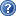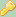# webassign hassignmentravneet001
List three methods of assigning probabilities. (Select all that apply.)• ## Show My Work

(Optional)You can submit show my work an unlimited number of times.(b) Find P(3 on 1st card and 10 on 2nd). (Enter your answer as a fraction.)
4/6634/663

(c) Find P(10 on 1st card and 3 on 2nd). (Enter your answer as a fraction.)
4/6634/663

(d) Find the probability of drawing a 10 and a 3 in either order. (Enter your answer as a fraction.)
8/6638/663

(b) How many sequences contain exactly two heads?

(b) age of a college professor chosen at random

(c) number of books in the college bookstore

(d) weight of a football player chosen at random

(e) number of lightning strikes in Rocky Mountain National Park on a given day

(b) Use a histogram to graph the probability distribution in part (a).
(c) Find the probability that a British nurse selected at random in 1851 would be 60 years of age or older. (Round your answer to three decimal places.)

(c) Interpret your results in the context of this problem.

(d) Find the sample size necessary for a 99% confidence level with maximal margin of error E = 3.00 for the mean plasma volume in male firefighters. (Round up to the nearest whole number.)

(d) Suppose the annual profits are 38000 per employee. How would you interpret this result by referring to the confidence interval you computed in part (b)?

• ## Show My Work

(Optional)• Posted: 4 years ago
• Due:
• Budget: \$10
Bids 0